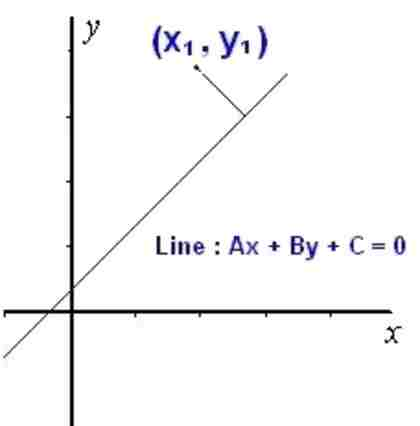### Perpendicular Length/Distance Calculator

Calculator finds the Perpendicular Length or Distance from a point to a line by putting the X and Y coordinates and the values for the given line (Ax+ By + C), very usefull tool for analytical geometry. The formula used as follows:
The distance from Ax+By+C=0 to P(x1,y1) is $$d = \frac{(A\,x_1 + B\,y_1 + C)}{\sqrt{A^2 + B^2}}$$Coordinates :- X1: Y1:
for Line : Ax + By + C = 0
 A: B: C:

 Length of Perpendicular

Example : The distance from line 3x+4y+5=0 to point P(3,4) is as follows $$d = \frac{(A\,x_1 + B\,y_1 + C)}{\sqrt{A^2 + B^2}}$$ $$= \frac{{3\,(3) + 4\,(4) + 5}}{\sqrt{3^2 + 4^2}}$$ $$= \frac{{9\, + 16\, + 5}}{\sqrt{9 + 16}}$$ $$= \frac{{30}}{\sqrt{25}}$$ $$= {6}$$